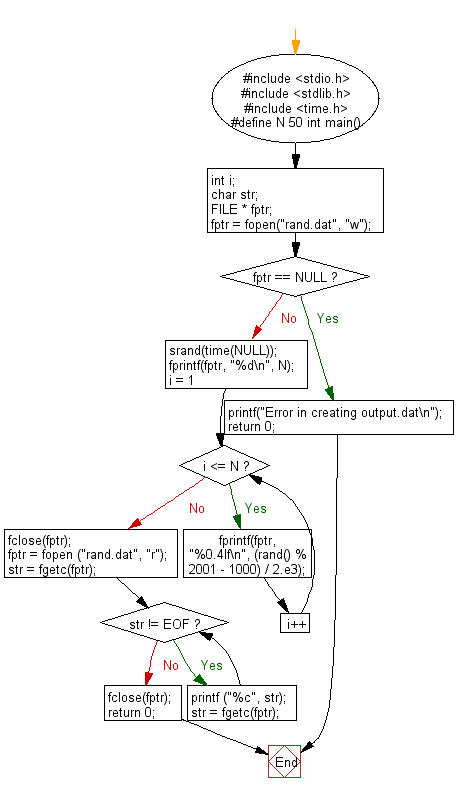﻿ C Program: Generates 50 random numbers between -0.5 and 0.5 - w3resource

# C Exercises: Generates 50 random numbers between -0.5 and 0.5

## C Basic Declarations and Expressions: Exercise-66 with Solution

Write a C program that generates 50 random numbers between -0.5 and 0.5 and writes them to the file rand.dat. The first line of ran.dat contains the number of random numbers, while the next 50 lines contain 50 random numbers.

Sample Solution:

C Code:

``````#include <stdio.h>
#include <stdlib.h>
#include <time.h>
#define N 50
int main() {
int i;
char str;
FILE * fptr;

// Open a file for writing
fptr = fopen("rand.dat", "w");
if (fptr == NULL) {
printf("Error in creating output.dat\n");
return 0;
}

// Seed the random number generator
srand(time(NULL));

// Write the number of values to the file
fprintf(fptr, "%d\n", N);

// Generate and write random numbers to the file
for (i = 1; i <= N; i++) {
fprintf(fptr, "%0.4lf\n", (rand() % 2001 - 1000) / 2.e3);
}

// Close the file
fclose(fptr);

// Open the file for reading
fptr = fopen ("rand.dat", "r");
str = fgetc(fptr);

// Print the contents of the file
while (str != EOF)
{
printf ("%c", str);
str = fgetc(fptr);
}

// Close the file
fclose(fptr);

return 0;
}
``````

Sample Output:

```50
-0.4215
0.2620
0.3065
-0.0485
-0.2085
-0.2490
-0.2780
0.2905
-0.3120
0.1275
0.4010
0.3060
0.4680
-0.1135
0.0130
-0.0145
-0.1890
-0.3825
0.3790
-0.2370
0.0840
-0.1985
0.2065
0.4445
0.0785
-0.2370
-0.0705
0.3870
-0.4695
0.1525
0.2755
0.3880
-0.3075
-0.1400
-0.3825
-0.0155
-0.1105
-0.1605
-0.4470
0.0780
0.4675
0.2330
-0.3380
0.2135
0.3980
0.1750
0.4780
-0.2915
0.0715
0.3565
```

Flowchart:C programming Code Editor:

What is the difficulty level of this exercise?

Test your Programming skills with w3resource's quiz.

﻿